# Program to check Niven Number Program in Java

In this tutorial you will learn about the Program to check Niven Number Program in Java and its application with practical example.

In this tutorial, we will learn to create a Java Program to check Niven Number Program in Java

## Prerequisites

Before starting with this tutorial we assume that you are best aware of the following Java programming topics:

• Java Operators.
• Basic Input and Output function in Java.
• Class and Object in Java.
• Basic Java programming.
• If-else statements in Java.
• Function in java.

A Number is called a Harshad number if sum of the digits is divisible by the sum of its digits

For example:-> 18 is a Harshad number base of 10, because sum of the its digit  which is “(1 and 8) = 9″ (1 + 8 = 9), and 18 is divisible by 9 (18 % 9 == 0).

## Program to check Niven Number Program in Java

In this program we would find the given number is Niven number first of all we will take the input from the user and check whether it is Niven number or not. If it’s not then the program will show the output as it’s not a Niven number.

# Niven number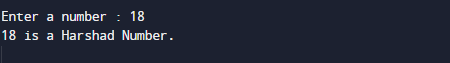# Not a Niven number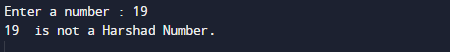In the above program, we have first declared and initialized a set variables required in the program.

• num= it will hold entered number.
• temp = it will hold temp value
• sum= for adding digit sum.
• d=  it will hold digits of a number

After declaring variables  we take a  number form user.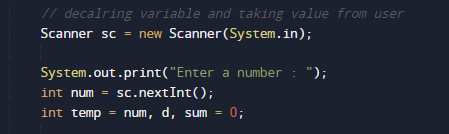After that we add digit sum of a number.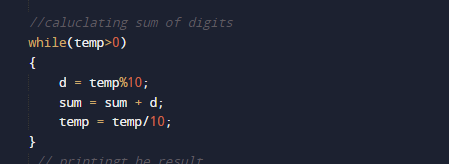and then we divide the sum with original number to find it is a Niven number or not.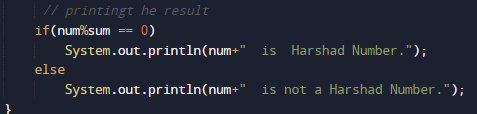Niven number (or harshad number)if it is divisible by the sum of its digits .

In this tutorial we have learn about the Program to check Niven Number Program in Java and its application with practical example. I hope you will like this tutorial.